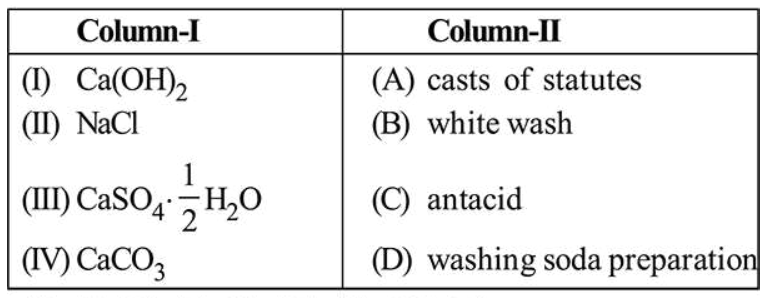# Match the following compounds (Column-I) with their uses (Column-II) :Question:

Match the following compounds (Column-I) with their uses (Column-II) :1. (I)-(D), (II)-(A), (III)-(C), (IV)-(B)

2. (I)-(B), (II)-(D), (III)-(A), (IV)-(C)

3. (I)-(B), (II)-(C), (III)-(D), (IV)-(A)

4. (I)-(C), (II)-(D), (III)-(B), (IV)-(A)

Correct Option: , 2

Solution:

(I) $\mathrm{Ca}(\mathrm{OH})_{2}$ is used in white wash.

(II) $\mathrm{NaCl}$ is used in preparation of washing soda $\left(\mathrm{Na}_{2} \mathrm{CO}_{3}\right)$.

(III) $\mathrm{CaSO}_{4} \cdot \frac{1}{2} \mathrm{H}_{2} \mathrm{O}$ is used for making casts of statues.

(IV) $\mathrm{CaCO}_{3}$ is used as an antacid.